# Partially ordered set

A partially ordered set (also called a poset) is a pair $$\langle P, \leq \rangle$$ of a set $$P$$ and a binary relation $$\leq$$ on $$P$$ such that for all $$p,q,r \in P$$, the following properties are satisfied:

$$P$$ is referred to as the poset’s underlying set and $$\leq$$ is referred to as its order relation. Posets are the central object of study in order theory.

# Notations and fundamentals

## Greater than

A greater than relation $$\geq$$ can be derived as the transpose of a poset’s less than relation: $$a \geq b$$ means that $$b \leq a$$.

## Incomparability

For poset elements $$p$$ and $$q$$, if neither $$p \leq q$$ nor $$q \leq p$$ then we say that $$p$$ and $$q$$ are incomparable, and write $$p \parallel q$$.

## Strict orders

From any poset $$\langle P, \leq \rangle$$, we can derive an underlying strict order $$<). For \(p, q \in P$$, $$p < q$$ means that the following conditions are satisfied:

• 1.) $$p \leq q$$

• 2.) $$p \neq q$$

## The cover relation

From any poset $$\langle P, \leq \rangle$$, we can derive an underlying cover relation $$\prec$$, defined such that for $$p,q \in P$$, $$p \prec q$$ whenever the following two conditions are satisfied:

• 1.) $$p < q$$

• 2.) For all $$r \in P$$, $$p \leq r < q$$ implies $$p = r$$

Simply put, $$p \prec q$$ means that $$q$$ is the smallest element of $$P$$ which is strictly greater than $$p$$. $$p \prec q$$ is pronounced “$p$ is covered by $$q$$”, or “$q$ covers $$p$$”, and $$q$$ is said to be a cover of $$p$$.

## Hasse diagrams

Let $$\langle P, \leq \rangle$$ be the poset such that $$P = \{ p, q, r \}$$ and $$\leq = \{(p,p),(p,q)(p,r),(q,q),(q,r),(r,r) \}$$. The order relation $$\leq$$, being binary, can be depicted as a directed graph, though the resulting image leaves something to be desired.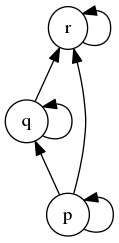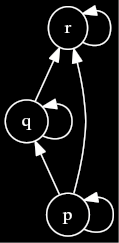comment: dot source:

digraph G { node = 0.1, height = 0.1 rankdir = BT; p → q; q → r; p → p; r → r; q → q; p → r; } <div>

Including an edge for every pair in $$\leq$$ results in a rather noisy looking graph. In particular, as long as we are under assumption that the graph we are viewing depicts a poset, placing a reflexive edge on each node is tedious and redundant. Our first step toward cleaning up this graph is to remove all reflexive edges, instead leaving reflexivity as an implicit assumption. Likewise, we can leave those edges which are implied by transitivity — $$(p,r)$$ is the only such edge in this poset — implicit. As a final step, we remove the arrow heads from our edges, leaving their directions implied by the y-coordinates of the nodes they connect: an edge between two nodes means that the poset element corresponding to the lower node is less than the poset element corresponding to the higher node. The simplified depiction of $$\langle P, \leq \rangle$$ then follows.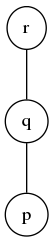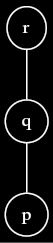comment: dot source:

digraph G { node = 0.1, height = 0.1; edge = “none”; rankdir = BT; p → q; q → r; } <div>

Such a depiction is called a Hasse diagram; drawing a Hasse diagram is the standard method for visually depicting a poset. Now that we understand what Hasse diagrams are, we can provide a concise definition: a Hasse diagram is a graph-based visual depiction of a poset $$\langle P, \leq \rangle$$, where elements of $$P$$ are represented as nodes, and for all $$(p,q) \in P^2$$ such that $$p \prec q$$, an upward edge is drawn from $$p$$ to $$q$$.

$$p \leq q$$ requires that $$p$$ must appear lower in a Hasse diagram than $$q$$. However, the converse is not true. In the following Hasse diagram, $$t \parallel r$$ even though $$t$$ is positioned lower than $$r$$.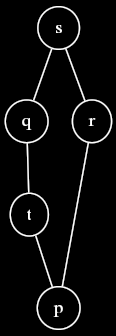comment: dot source:

digraph G { node = 0.1, height = 0.1; edge = “none”; rankdir = BT; p → t; p → r; t → q; q → s; r → s; } <div>

## Duality

Every poset $$\langle P, \leq \rangle$$ has a corresponding dual poset $$\langle P^{\partial}, \geq \rangle$$, where $$P^{\partial}$$ (pronounced “P op”) is a set whose elements are in correspondence with those of $$P$$, and $$\geq$$ is the transpose of $$\leq$$ discussed previously. The Hasse diagram of $$P^{\partial}$$ is obtained by flipping the Hasse diagram of $$P$$ upside down. Whenever $$\phi$$ is a propsition about posets, we obtain $$\phi$$’s dual proposition $$\phi^{\partial}$$ by replacing all occurences of $$\leq$$ with $$\geq$$ and all occurrences of $$\geq$$ with $$\leq$$.

The existence of dual posets and dual propositions gives rise to the duality principle: if a proposition $$\forall P. \phi$$, quantified over all posets, is true then its dual $$\forall P. \phi^{\partial}$$ is also true. The duality principle works because instantiating $$\forall P. \phi$$ with a poset $$P$$ is equivalent to instantiating $$\forall P. \phi^{\partial}$$ with $$P^{\partial}$$. This is due to the fact that $$a \leq b$$ in $$P$$ iff $$a \geq b$$ in $$P^{\partial}$$. Theorems involving posets often come with a free dual theorem thanks to the duality principle.

knows-requisite(Category theory):

# Poset as category

Any poset $$\langle P, \leq \rangle$$ can be viewed as a category in which the objects are the elements of $$P$$, and for all $$p,q \in P$$, there is a unique morphism from $$p$$ to $$q$$ iff $$p \leq q$$. This category has closure under morphism composition due to the transitivity of $$\leq$$ and identity morphisms due to the reflexivity of $$\leq$$. Associativity of morphism composition stems from the uniqueness of arrows between any pair of objects. The functors between poset categories are monotone maps. <div>

For some additional examples of posets, see Poset: Examples. To test your knowledge of posets, try these exercises. The most useful operators on elements of a poset are called the join and meet. We can relate the elements of one poset to another through the use of monotone functions.

Children:

Parents:

• Order theory

The study of binary relations that are reflexive, transitive, and antisymmetic.

• @1 I was going to add an examples lens to this page, but I seem to have lost the ability to create lenses. I remember being able to create lenses by placing an orange button in the bottom right corner. That does not currently work, however: the “create lens” icon doesn’t show up.

• We removed that button from the quick menu because it had too many buttons. Now you have to create a page, then add this page as a parent, and then change the page’s type to “Lens” in settings. As I typed this, I realized how many steps that is. We’ll make this simpler.

• Should the p’s and q’s in one of these be switched?

• Would it be appropriate to link to the Underlying set page here?

• Maybe. I haven’t done so because the underlying set page describes underlying sets specifically in terms of algebraic structures. I think that a link to that page would therefore just cause confusion.

• describes underlying sets specifically in terms of algebraic structures

Yeah, I was wondering about that. Does it make sense to have an “underlying set” page that works for both cases?

Actually, I’m going to ask this in the slack, as others may have opinions…

• I’m thinking the full name of the article should be “Partially ordered set”, with “poset” as an alias and alternate form in the article.